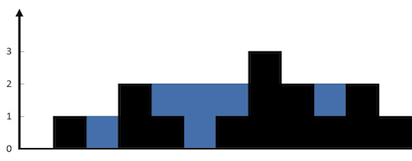# 接雨水

给定 n 个非负整数表示每个宽度为 1 的柱子的高度图，计算按此排列的柱子，下雨之后能接多少雨水。输入: [0,1,0,2,1,0,1,3,2,1,2,1]



(1)  找到最大的位置，然后进行左右移动。

在找到最大的高度之前，设置一个左边的围栏， 左边的一定可以找到比左边围栏高的围栏，所以，只要碰到比自己低的就，记录一下水的深度，一直到比自己高的为止，然后重新记录左边的围栏。一直到最高的位置为止。

右边也是可以这样的。 一直到最高的围栏为止。

class Solution {
public:
int trap(vector<int>& height) {
long long Result=0;
int Size=height.size();
if(Size==0)
return 0;
int Begin=height;
int water=0;
int IndexOfMax=0;
for(int i=1;i<Size;i++){
if(height[i]>height[IndexOfMax])
IndexOfMax=i;
}
for(int i=1;i<=IndexOfMax;i++){
if(height[i]>=Begin)
{
Result +=water;
Begin =height[i];
water =0;
}else{
water +=Begin-height[i];
}
}
water=0;
Begin=height[Size-1];
for(int i=Size-2;i>=IndexOfMax;i--){
if(height[i]>=Begin)
{
Result +=water;
Begin =height[i];
water =0;
}else{

water +=Begin-height[i];
}
}
return Result;
}
};

（2）用栈去做

class Solution {
public:
int trap(vector<int>& height) {
int Result=0;
int SIZE=height.size();
stack<int> st;
for(int i=0;i<SIZE;i++)
{
while(!st.empty() && height[i]>=height[st.top()])
{
int buttomIndex=st.top();
st.pop();
if(st.empty())
break;
int distance=i-st.top()-1;
int waterlin=min(height[i],height[st.top()])-height[buttomIndex];
Result +=waterlin*distance;
}
st.push(i);
}
return Result;
}
};

10-09
03-0715306-28584
01-25974
10-01332
09-157477